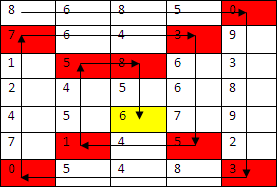### Problem Statement

You are playing a game where you must traverse a rectangular grid of cells using a spiral path. The map is given in a String[] levelMap, where the j-th character of the i-th element is the number of points associated with the cell in row i, column j. Rows are numbered from top to bottom, starting at 0, and columns are numbered from left to right, starting at 0. All coordinates in this problem will be given as (row, column).

You start at cell (0,0), the top left corner of the grid. You are facing right. You move by repeating the following strategy until you have visited every single cell on the grid exactly once. If there is an adjacent cell in front of you that you haven't visited yet, move forward to that cell. Otherwise, if there are still unvisited cells on the grid, turn 90 degrees clockwise.

To calculate your final score, add up all the points for the cells that you visited, but don't include the cells in which you changed direction. The first and last cells in your path will always be included in your final score.

See examples for further clarification.

### Definition

 Class: SpiralWalking Method: totalPoints Parameters: String[] Returns: int Method signature: int totalPoints(String[] levelMap) (be sure your method is public)

### Constraints

-levelMap will contain between 2 and 50 elements, inclusive.

-All elements of levelMap will contain the same number of characters.

-Each element of levelMap will contain between 2 and 50 digits ('0'-'9'), inclusive.

### Examples

0)

 ```{"111", "111", "111"}```
`Returns: 5`
 This is the spiral path you must follow: (0,0) -> (0,1) -> (0,2) -> (1,2) -> (2,2) -> (2,1) -> (2,0) -> (1,0) -> (1,1).
1)

 ```{"101", "110"}```
`Returns: 3`
 The grid is not always a square.
2)

 ```{"00", "10"}```
`Returns: 1`
3)

 ```{"86850", "76439", "15863", "24568", "45679", "71452", "05483"}```
`Returns: 142`
 The following image shows your path. The yellow cell is the last cell you visit. You receive points for all the cells except the red ones.#### Problem url:

http://www.topcoder.com/stat?c=problem_statement&pm=9793

#### Problem stats url:

http://www.topcoder.com/tc?module=ProblemDetail&rd=12179&pm=9793

Gluk

#### Testers:

PabloGilberto , Olexiy , ivan_metelsky , ged

Simulation